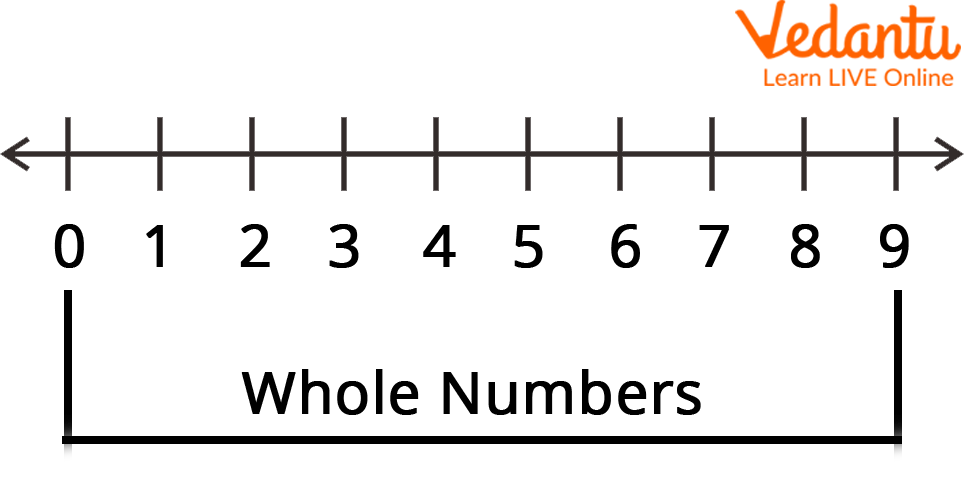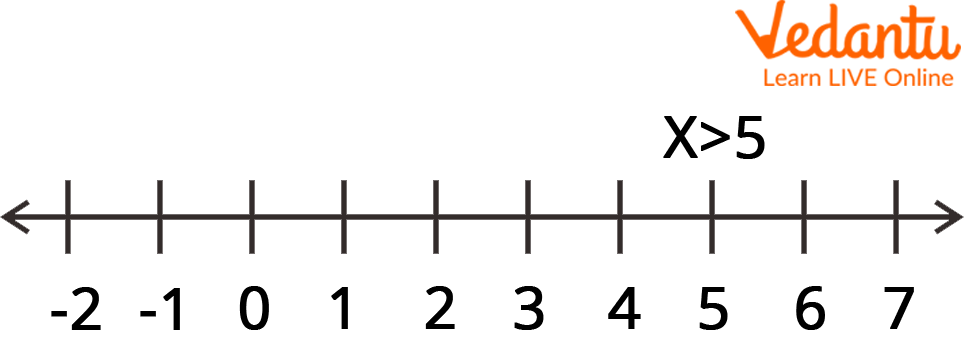Courses
Courses for Kids
Free study material
Free LIVE classes
More

# Representation on a Number lineLIVE
Join Vedantu’s FREE Mastercalss

## How to Represent on the Number Line?

A straight line of numbers is shown visually as a number line. Here, the number line is useful for comparing integers on an endless line that extends on both sides i.e. horizontally or vertically, at equal intervals.

As we walk towards the right side of a horizontal number line, the numbers grow; as we move towards the left, the numbers drop. A number line is a line that has an endlessly long list of positive and negative integers on either side of a point called "zero" in the middle. A Maths student can use a number line as an example to solve addition and subtraction problems. We will understand here what every point on a number line represents.

## What Is a Number Line?

A number line is a visual representation of numbers on a straight line drawn horizontally or vertically. When we write numbers on a number line, we can easily compare them and execute simple arithmetic operations on them. A number line's origin is defined as zero (0). The numbers to the left of 0 are all negative, whereas the numbers to the right of 0 are all positive. So, as we walk towards the right on a number line, the value of numbers increases. This signifies that the numbers on the right are more than those on the left. For instance, 3 comes after 1, hence 3 > 1.

## Represent on a Number Line

The visual representation of numbers on a straight line is known as a number line. A straight line is divided into several segments, each with a unit separation. We can quickly distinguish between two numbers and do comparisons between them by expressing them on a number line. It can alternatively be described as a line with numerals marked at regular intervals. It demonstrates elementary mathematical procedures with ease.

The Steps to Represent on a Number Line are as Follows:

• Make a clean line.

• Put a zero at the very leftmost spot.

• Place a point just to the right of zero. Indicate 1, 2, 3, etc. These marks must be spaced evenly apart.Whole Number from 0 to 9 on Number Line

## Every Point on a Number Line Represents a Number

Each point on a number line represents a specific number i.e. a unique real number. It can represent any real number, including natural and whole numbers. Recall that the natural number is the collection of all counting numbers, i.e., 1, 2, 3, 4, 5, 6, etc., but the whole number is a collection of numbers that contains all counting numbers, i.e., 1, 2, 3, 4, 5, 6, etc., as well as "zero (0)." Real numbers, "such as natural numbers, whole numbers, integers, rational numbers, and irrational numbers," are compared by using the number line.

## Solved Examples

Q 1. Put x greater than 5 on a number line.

Ans: We will use the steps listed below to represent x > 5 on a number line:

• Draw a number line, mark 0, and then draw equal distances to the right and left, as shown below.

• The value of x in the inequality x > 5 is bigger than 5. Consequently, we must go to the right of 5.

• Draw a black empty circle as indicated in the figure. Shade the line to the right side until it reaches the arrow. Since a blank circle indicates that a point is not included, the circle at point 5 will be left unfilled. This indicates that x is not equal to and is greater than 5.Number Line

## Practice Questions

Q 1. Put x greater than or equal to 8 on a number line.

Q 2. Put x in fraction i.e. \$\dfrac{7}{5}\$ on a number line.

## Summary

The horizontal straight lines in Mathematics known as number lines are where integers are arranged in equal intervals. A number line can be used to represent every number in a sequence. Here, the endpoints of this number line go forever. Integers are arranged on a number line.

A number line is exactly what it sounds like—a horizontal, straight line with numbers spaced evenly apart throughout its length. Since it's not a ruler, the distance between each number is irrelevant; instead, the numbers on the line specify how it should be used. The vertical equivalent of a number line is a number ladder.

Last updated date: 22nd Sep 2023
Total views: 109.8k
Views today: 3.09k

## FAQs on Representation on a Number line

1. What is the origin of the number line?

Although the idea of a number line originally appeared in the writings of Cantor and Dedekind, the phrase itself has been in use since 1912 under the names "number scale" and "number line". Therefore, a complete scale extending in both directions from zero is formed by the positive and negative numbers together. You must place labelled points on the number line in some number line problems. The sketching tools below the number line are inactive for these questions and a series of labelled points are shown above the number line instead.

2. What is the number line's scale?

The logarithmic scale, which represents positive integers on a line so that the distance between any two points is equal to one unit of length, is one of the most popular options if the ratio of the depicted numbers has a fixed value, usually 10. Now, integers are represented and marked uniform spaced dots on a number line which includes all real numbers going in both directions, even though this image only displays the integers from -9 to 9, as well as numbers that are in between the integers. Here, it is frequently employed as a teaching tool for basic addition and subtraction, particularly when negative nos. are involved.

3. Does the number line represent a circle?

In reality, a number line is a geometric model where the points stand in for numbers. Since the real numbers are unbounded at "both ends," a straight line makes a better model of them than a circle does.